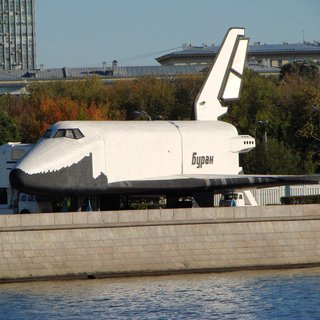OpenCV  3.1.0 Open Source Computer Vision

## Introduction

In this tutorial you will learn how to use opencv_dnn module for image classification by using GoogLeNet trained network from Caffe model zoo.

We will demonstrate results of this example on the following picture.Buran space shuttle

## Source Code

We will be using snippets from the example application, that can be downloaded here.

#include <opencv2/dnn.hpp>
using namespace cv;
using namespace cv::dnn;
#include <fstream>
#include <iostream>
#include <cstdlib>
using namespace std;
/* Find best class for the blob (i. e. class with maximal probability) */
void getMaxClass(dnn::Blob &probBlob, int *classId, double *classProb)
{
Mat probMat = probBlob.matRefConst().reshape(1, 1); //reshape the blob to 1x1000 matrix
Point classNumber;
minMaxLoc(probMat, NULL, classProb, NULL, &classNumber);
*classId = classNumber.x;
}
std::vector<String> readClassNames(const char *filename = "synset_words.txt")
{
std::vector<String> classNames;
std::ifstream fp(filename);
if (!fp.is_open())
{
std::cerr << "File with classes labels not found: " << filename << std::endl;
exit(-1);
}
std::string name;
while (!fp.eof())
{
std::getline(fp, name);
if (name.length())
classNames.push_back( name.substr(name.find(' ')+1) );
}
fp.close();
return classNames;
}
int main(int argc, char **argv)
{
String imageFile = (argc > 1) ? argv : "space_shuttle.jpg";
try //Try to import Caffe GoogleNet model
{
importer = dnn::createCaffeImporter(modelTxt, modelBin);
}
catch (const cv::Exception &err) //Importer can throw errors, we will catch them
{
std::cerr << err.msg << std::endl;
}
if (!importer)
{
std::cerr << "Can't load network by using the following files: " << std::endl;
std::cerr << "prototxt: " << modelTxt << std::endl;
std::cerr << "caffemodel: " << modelBin << std::endl;
exit(-1);
}
dnn::Net net;
importer->populateNet(net);
importer.release(); //We don't need importer anymore
if (img.empty())
{
std::cerr << "Can't read image from the file: " << imageFile << std::endl;
exit(-1);
}
resize(img, img, Size(224, 224)); //GoogLeNet accepts only 224x224 RGB-images
dnn::Blob inputBlob = dnn::Blob(img); //Convert Mat to dnn::Blob image batch
net.setBlob(".data", inputBlob); //set the network input
net.forward(); //compute output
dnn::Blob prob = net.getBlob("prob"); //gather output of "prob" layer
int classId;
double classProb;
getMaxClass(prob, &classId, &classProb);//find the best class
std::cout << "Best class: #" << classId << " '" << classNames.at(classId) << "'" << std::endl;
std::cout << "Probability: " << classProb * 100 << "%" << std::endl;
return 0;
} //main

## Explanation

Also you need file with names of ILSVRC2012 classes: synset_words.txt.

Put these files into working dir of this program example.

2. Create the importer of Caffe models
Ptr<dnn::Importer> importer;
try //Try to import Caffe GoogleNet model
{
importer = dnn::createCaffeImporter(modelTxt, modelBin);
}
catch (const cv::Exception &err) //Importer can throw errors, we will catch them
{
std::cerr << err.msg << std::endl;
}
3. Create the network and initialize its by using the created importer
dnn::Net net;
importer->populateNet(net);
importer.release(); //We don't need importer anymore
4. Read input image and convert to the blob, acceptable by GoogleNet

if (img.empty())
{
std::cerr << "Can't read image from the file: " << imageFile << std::endl;
exit(-1);
}
resize(img, img, Size(224, 224)); //GoogLeNet accepts only 224x224 RGB-images
dnn::Blob inputBlob = dnn::Blob(img); //Convert Mat to dnn::Blob image batch

Firstly, we resize the image and change its channel sequence order.

Now image is actually a 3-dimensional array with 224x224x3 shape.

Next, we convert the image to 4-dimensional blob (so-called batch) with 1x2x224x224 shape by using special cv::dnn::Blob constructor.

5. Pass the blob to the network

net.setBlob(".data", inputBlob); //set the network input

In bvlc_googlenet.prototxt the network input blob named as "data", therefore this blob labeled as ".data" in opencv_dnn API.

Other blobs labeled as "name_of_layer.name_of_layer_output".

6. Make forward pass
net.forward(); //compute output
During the forward pass output of each network layer is computed, but in this example we need output from "prob" layer only.
7. Determine the best class
dnn::Blob prob = net.getBlob("prob"); //gather output of "prob" layer
int classId;
double classProb;
getMaxClass(prob, &classId, &classProb);//find the best class
We put the output of "prob" layer, which contain probabilities for each of 1000 ILSVRC2012 image classes, to the prob blob. And find the index of element with maximal value in this one. This index correspond to the class of the image.
8. Print results